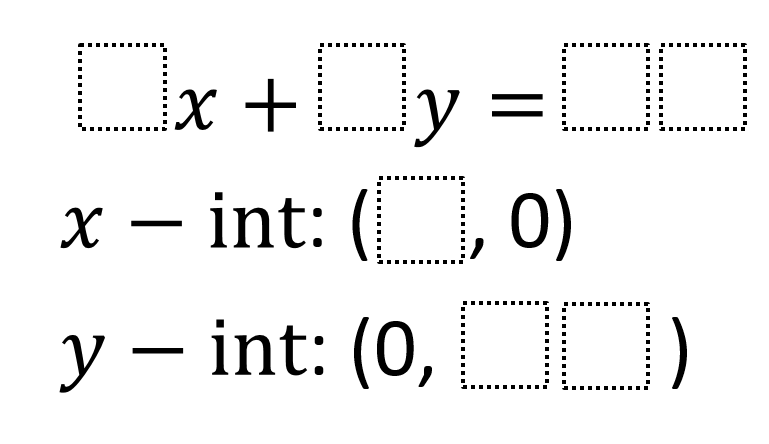Home > High School: Functions > Intercept Form Equations

# Intercept Form Equations

Directions: Using digits 1-9, write an equation of a line in standard form with given x- and y-intercepts. Each number can only be used at most once.### Hint

What numbers can we rule out as coefficients for x and y in the equation?

### Answer

Possible answer:
9x + 2y = 36
x-intercept: (4,0)
y-intercept: (0,18)

Source: Andy Schwen

## Creating Sequences

Directions: Using the digits 0-9, at most one time each, complete the first three terms …